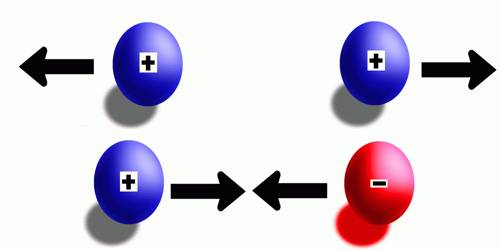Physics

# Electromagnetic ForceElectromagnetic Force: The force between two charges is called electric force and that between two magnets is called magnetic force. But electric force and magnetic force are closely related to each other. The electromagnetic force is the combination of electric forces and magnetic forces. The electromagnetic force causes electric and magnetic effects such as the repulsion between like electrical charges or the interaction of bar magnets. It is long-ranged but much weaker than the strong force. Virtually the force acting between two charged particles in motion is the electromagnetic force. When charged particles are in motion, they create a magnetic field. On the other hand, varying magnetic field acts as the source of the electric field. Scientists think that due to the mutual exchange of massless, charge fewer particles called photons this force becomes effective. It can be attractive or repulsive and acts only between pieces of matter carrying an electrical charge. Electricity, magnetism, and light are all produced by this force.

Apart from gravitational force, all forces that we can experience directly as a push or pull are electromagnetic in nature. Frictional force, contact force, elastic force, chemical force etc involve electromagnetic force. It can be attractive or repulsive and acts only between pieces of matter carrying an electrical charge. Electricity, magnetism, and light are all produced by this force.

In the universe, there exists an attraction force between any two particles or objects. This force is called gravitational force. The magnitude of this force is proportional to the product of masses of due two objects or particles and inversely proportional to the square of the distance between them. Scientists believe that particles, called gravitons, are exchanged between the bodies which are responsible for gravitational attraction between the bodies.